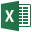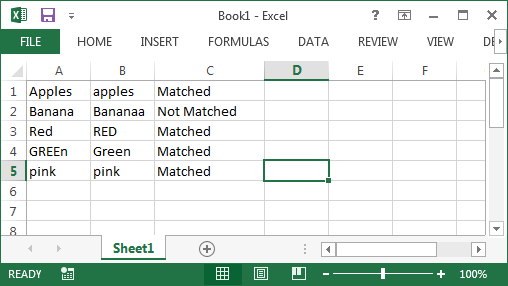### Description:

We can automate task to Change Text Case – Upper Lower in Excel VBA. See the following examples to know how to do this.

### Change Text Case – Upper Lower in Excel VBA: SolutionWe can use UCase and LCase function to change a text into Upper and Lower.

### Examples

Following are the examples to show you how to do this practically.

```Sub sbChangeCASE()
'Upper Case
Range("A3") = UCase(Range("A3"))

'Lower Case
Range("A4") = LCase(Range("A4"))
End Sub
```
###### Instructions:

Follow the below instructions to do it yourself.

1. Open an excel workbook
2. Add some text at A3 and A4 ranges
3. Press Alt+F11 to open VBA Editor
4. Insert a Module for Insert Menu
5. Copy the above code and Paste in the code window
6. Save the file as macro enabled workbook
7. Press F5 to execute it
###### Practical Applications:

Most of the times we use UCASE and LCASE function while comparing the text. For examples if we have two Columns and want to compare the Cells of each columns to check if they are same.

Let’s assume the following data is there in Column A and B, and we want to compare it and print it in Column C.

A B
Apples apples
Banana Bananaa
Red RED
GREEn Green
pink pink

If you write a procedure to compare this data without using UCASE or LCASE function, we may not get the desired results.

See the following Example code and output. We can observe that the its comparing but its case sensitive.

```Sub sbCompareColumns_1()
iCntr = 1
Do While Cells(iCntr, 1) <> ""

If Cells(iCntr, 1) = Cells(iCntr, 2) Then
Cells(iCntr, 3) = "Matched"
Else
Cells(iCntr, 3) = "Not Matched"
End If

iCntr = iCntr + 1
Loop
End Sub
```

You can see the output in Column C:

I am using the same code with UCASE function, so that first it will convert to uppercase then compare it. We can avoid the case sensitivity.

```
Sub sbCompareColumns_2()
iCntr = 1
Do While Cells(iCntr, 1) <> ""

If UCase(Cells(iCntr, 1)) = UCase(Cells(iCntr, 2)) Then
Cells(iCntr, 3) = "Matched"
Else
Cells(iCntr, 3) = "Not Matched"
End If

iCntr = iCntr + 1
Loop
End Sub
```

You can see the output in Column C is different than the above result.120+ Professional Project Management Templates!

A Powerful & Multi-purpose Templates for project management. Now seamlessly manage your projects, tasks, meetings, presentations, teams, customers, stakeholders and time. This page describes all the amazing new features and options that come with our premium templates.

LIMITED TIME OFFER
ON SALE85% OFF

Excel Templates

120+ Project Management Templates Pack

Excel | PowerPoint | Word

ULTIMATE RESOURCE MANAGEMENT TEMPLATE

Excel Template

50+ Essential Project Management Templates

Excel | PowerPoint | Word

Project Portfolio Management Templates

Excel | PowerPoint Templates

50+ Excel Project Management Templates

Excel Templates

By Published On: February 9th, 2013Categories: Excel VBATags:

Excel VBA Developer having around 8 years of experience in using Excel and VBA for automating the daily tasks, reports generation and dashboards preparation. Valli is sharing to helps us automating daily tasks.

1. gcpath March 2, 2014 at 2:26 AM

i tried the same but i am getting error as “variable not defined”

• gcpath March 2, 2014 at 2:30 AM

tried Dim iCntr it worked

thanksss…

2. Immanuel March 19, 2014 at 9:39 PM

When I copy the below code in to the VB editor .. the syntax lines come up as red , and pops up an error
Sub sbChangeCASE()
‘Upper Case
Range("A3") = UCase(Range("A3"))

‘Lower Case
Range("A4") = LCase(Range("A4"))
End Sub

• PNRao March 20, 2014 at 1:27 AM

Please try this, the single quote is for commenting the lines, you can remove it.
``` Sub sbChangeCASE() 'Upper Case Range("A3") = UCase(Range("A3"))```

``` 'Lower Case Range("A4") = LCase(Range("A4")) End Sub ```

Thanks-PNRao!

3. immanuel March 21, 2014 at 8:05 PM

Hi,
Is iCntr the same this as inStr ….returning value of the occurring string..
I don’t quite get the below script

Sub sbCompareColumns_1()
iCntr = 1
Do While Cells(iCntr, 1) “”
If Cells(iCntr, 1) = Cells(iCntr, 2)
Then Cells(iCntr, 3) = “Matched”
Else Cells(iCntr, 3) = “Not Matched”
End If
iCntr = iCntr + 1
LoopEnd
Sub

• PNRao March 22, 2014 at 12:49 AM

Hi,
Please see the explained code below:
``` Sub sbCompareColumns_1() iCntr = 1 'this will loop until the the Column A is blank Do While Cells(iCntr, 1) <> "" 'Here it is comparing the values If Cells(iCntr, 1) = Cells(iCntr, 2) Then Cells(iCntr, 3) = "Matched" Else Cells(iCntr, 3) = "Not Matched" End If 'moving to next cell iCntr = iCntr + 1 Loop End Sub ```

Thanks-PNRao!

4. Sitharth May 6, 2015 at 5:09 PM

Thanks -PNRao……

5. Bhukailas October 24, 2016 at 4:25 PM

Is there any short cut to toggle case of entered text in Cells.

6. Yogesh August 22, 2018 at 4:16 PM

Hello its very Nice if possible to give me this code for 2 different sheet compare and result in sheet 1
Sub sbCompareColumns_1()
iCntr = 1
Do While Cells(iCntr, 1) “”

If Cells(iCntr, 1) = Cells(iCntr, 2) Then
Cells(iCntr, 3) = “Matched”
Else
Cells(iCntr, 3) = “Not Matched”
End If

iCntr = iCntr + 1
Loop
End Sub

please above code convert into 2 different sheet its very helpful for me